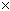SEARCH HOMEMath Central Quandaries & QueriesQuestion from Naseer, a student: I read somewhere that prime number can be a factor of square only if it occurs atleat twice in its square please explian this with examples. thank you for your timeHello,

Very loosely (not a rigorous proof) if a number is a square, say n = zz and a prime p divides it, what do you think about p dividing z?

If p didn't divide z but divides n this would imply 'part' of p divided one of the z's and the 'rest' of p divided the other. However p is pime and can't be broken into parts thus p divides both z's and p2 divides n.

If you're familiar with the canonical representation of an integer n as a product of primes then the truth of your statement is obvious.

Penny

(editor's note) This is often called the "Fundamental Theorem of Arithmetic".Math Central is supported by the University of Regina and The Pacific Institute for the Mathematical Sciences.